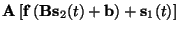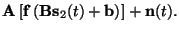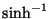Next: Cost function Up: Multi-Layer Perceptrons as Previous: Ensemble learning

# Model structure

The basic model structure is the ordinary MLP network. It has a slight improvement, however, and to motivate this, let us first consider how the learning with generative models looks like in practice.

As usually, we start with a random initialisation of the weights. Then we take the first observed data vector and find those values of the latent variables which best explain the observed data. In vector quantisation, for instance, this is very easy. The latent variable is the index of the model vector which is used for representing the data, and therefore the best value for the latent variable is simply the index of the closest model vector.

Inverting an MLP network is harder, however, and we are going to use gradient descent for doing it. Usually back propagation is used for updating the weights, but it can be used for adapting the unknown inputs, the latent variables as well. Typically the gradient descent has to be iterated several times in order to find the optimal latent variables.

To put the same thing differently, in supervised learning we are presented with the inputs and desired outputs. In our case the latent variables play the role of inputs and the observations play the role of outputs. The difference is that the latent variables are unknown and to find them, the model has to be inverted.

Once the optimal latent variables are found, the inputs of the MLP network are known and the learning proceeds as in supervised learning: the weights are adapted so as to make the mapping from the found latent variables to the observed data even better. Again we can draw a parallel from vector quantisation: in most learning algorithms the best matching model vector is moved even closer to the input.

Then we take the next data vector, find the latent variables that best describe the data by iterating the gradient descent a few times, adapt the weights, and so on.

In the beginning the learning can be slow, however. When the model is random, no values of the latent variables are able to explain much of the data. The optimal latent variables can be more or less random, and without sensible inputs it is difficult to adapt the mapping. It would therefore seem that learning is bound to be slower with unsupervised generative models than with supervised models.

Luckily, it turns out that the situation is much better because the mapping can be learned layer by layer starting from the layers closest to the observations. The point is to create parts of the network only when they may have meaningful input. In this process the model is refined when getting closer to the solution.

In the beginning, only the linear layer is created, together with first layer latent variables which act as training wheels for the network. At this point the mapping is linear and the network quickly finds some meaningful values for the first layer weights.

After the first layer has found a rough representation, the second, nonlinear layer is added on top of the first layer. Since the first layer weights already have reasonable values, the second layer learns much faster. Initially the data is represented mainly by the first layer latent variables, but gradually the second layer latent variables take over and the first layer latent variables become silent.

We can now formalise the model used in this work. Let x(t)denote the observed data vector at time t; s1(t) and s2(t) the vectors of latent variables of the first and the second layer at time t; A and B the matrices containing the weights on the first and the second layer, respectively; b the vector of biases for the second layer and f the vector of nonlinear activation functions. As all real signals contain noise, we shall assume that observations are corrupted by Gaussian noise denoted by n(t). Using this notation, the model for the observations passes through the three phases described below:

 x(t) = A s1(t) + n(t) (2) x(t) =+ n(t) (3) x(t) =(4)

The nonlinearity most often used in MLP networks is the hyperbolic tangent, but it has a disadvantage from the point of view of this application. It saturates for large values of its inputs which can cause problems during the inversion of the model. For the sake of numerical stability, the inverse hyperbolic sine,, is chosen instead. It is also a sigmoidal function but instead of saturating it behaves logarithmically for large values.

The latent variables are assumed to be independent and Gaussian. The independence assumption is natural as the goal of the model is to find the underlying independent causes of the observations. If the latent variables were dependent, then they would presumably have a common cause which should be modelled by yet another latent variable.

Even the Gaussianity assumption is usually not unrealistic. The network has nonlinearities which can transform the Gaussian distributions to virtually any other regular distribution. This is why with linear models it makes a difference whether the latent variables are assumed to have Gaussian, as in PCA, or non-Gaussian distributions, as in ICA, but for nonlinear models these assumptions do not make such a great difference. It may, of course, sometimes be that an explicit model of a non-Gaussian distribution, e.g., by mixtures of Gaussians as in , is simpler than an implicit model with nonlinearities.

The parameters of the network are: (1) the weight matrices Aand B and the vector of biases b; (2) the parameters of the distributions of the noise, latent variables and column vectors of the weight matrices; and (3), hyperparameters which are used for defining the distributions of the biases and the parameters in the group (2). For simplicity, all the parametrised distributions are assumed to be Gaussian.

This kind of hierarchical description of the distributions of the parameters in the model is a standard procedure in probabilistic modelling. Its strength is that knowledge about equivalent status of different parameters can be easily incorporated. All the variances of the noise components, for instance, have a similar status in the model and this is reflected by the fact that their distributions are assumed to be governed by common hyperparameters. Often there is some vague prior information about the distributions of the hyperparameters, but the amount of information is, in any case, very small compared to the amount of information in the data. Here the hyperparameters are assigned flat priors.Next: Cost function Up: Multi-Layer Perceptrons as Previous: Ensemble learning
Harri Lappalainen
1999-05-25